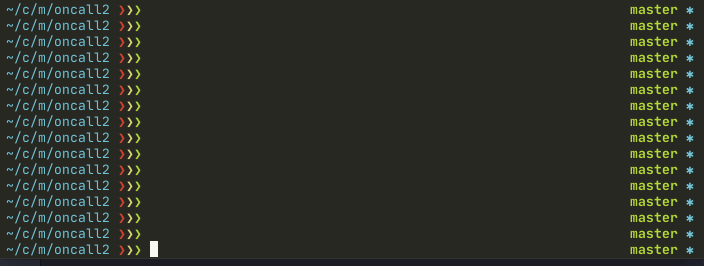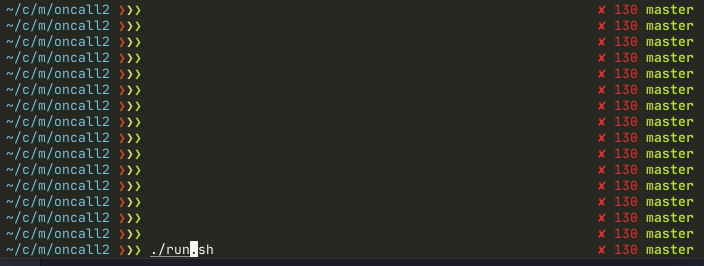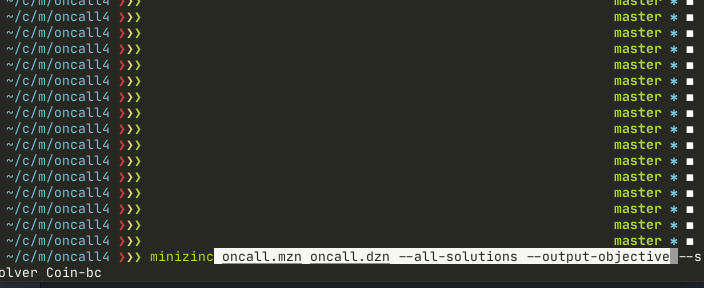# Discrete optimization for on-call scheduling. Part 1

August 29, 2020

The engineering team of RainforestQA (YC S12) is remote and distributed around the globe, with developers in America, Europe, and Asia. Our working hours cover almost all time-zones, about 22 hours from full time-zone coverage. Yet for distributing our on-call schedule, we were unhappy with PagerDuty’s standard daily rotation. ​​For a distributed team, why does somebody need to be on-call at night when other team members are working right now?

## The Tyrant

Starting in 2017, a new internal project for on-call scheduling was born - the Tyrant - as tool to handle On-Call scheduling across time-zones.

Over time the project evolved - scheduling algorithm and technology. In 2019, during a hackathon session in Kuala Lumpur (learn how to run off-sites for distributed teams), the latest version created with discrete optimization as its core.

Tyrant runs as a cron job every Saturday. It gets hour’s preferences from developers, builds on-call schedule, and pushes it to PagerDuty ​​plus emails to folks.

This series of blog posts will describe all the technical details about using discrete optimization for building schedule.## What is Discrete Optimization

Discrete optimization is the selection of a best element (answer) with regard to some criterion, and where some of the variables are restricted to be discrete. For practical purposes it is a DSL. A user models the problem in specific language, and solvers finds the best answer.

I had used discrete optimization for a few small hobby projects and for programming challenges. But for a long time I could not find a use in day-to-day jobs; every time I had a project solvable by discrete optimization - some naive algorithms always beat it. Sometimes by speed; sometimes better quality over naive algorithms was not worth paying by extra complexity.

Tyrant is a completely different story. As it creates the schedule only once per week, speed isn’t important - the quality of the result is.Tyrant’s core is implemented in MiniZinc and the first 3 blog posts will cover it. MiniZinc is a high-level modelling language and compiler. MiniZinc compiles into FlatZinc, a low-level language that is understood by a wide range of solvers (an implementation of a discrete optimization algorithm). For us it means faster development, self-explanatory model, and ability to switch solvers.In Part 4, we’ll look inside MiniZinc by manually translation an on-call model to low-level mixed integer format PIP-format, and then solve the problem with SCIP solver.

### Introduction to MiniZinc

Let’s explore a simple problem to familiarize ourselves with the basic MiniZinc syntax. Imagine a small car factory named “BetterThanTesla”. You have 100 lithium batteries, and 190000 kilograms of metal. Building a “Cybertruck” requires 1 battery and 2500 kg of metal, while building “Model3” requires also 1 battery, but only 1500 kg of metal. You would like to know how many of each cars to produce to maximize profit if you can sell “Cybertruck” for \$40k and “Model3” for \$35k.

``````int: total_nb_battery = 100;  % fixed, input parameters
int: total_kg_metal = 190000; % fixed, input parameters

% variable, possible number of different cars
var 0..total_nb_battery: nb_cybertruck;
var 0..total_nb_battery: nb_model3;

var int: profit = nb_cybertruck * 40000 + nb_model3 * 35000;

% limit total number of kilograms
constraint nb_cybertruck * 2500 + nb_model3 * 1500 <= total_kg_metal;
% limit total number of batteries
constraint nb_cybertruck + nb_model3 <= total_nb_battery;

solve maximize profit;
``````

The amount of words to describe the problem in English would be about the same as in MiniZinc. The code is self-explanatory even without knowing a lot about the syntax.

There’s a few nuances:

• `int` (first 2 lines) fixed integer variables - value should be defined
• `var int` unfixed integer variable - MiniZinc figures out the value later
• `constraint` some expression that should be true

The line: `var 0..total_nb_battery: nb_model3;` says variable `nb_model3` should be an integer from 0 to total_nb_battery. This could be written as:

``````var int: nb_model3;
constraint nb_model3 >= 0;
constraint nb_model3 <= total_nb_battery;
``````

The last line `solve maximize profit` tells MiniZinc what to optimize.

If you run: `minizinc hello1.mzn --output-objective`, you should get how many cars to build in order to maximize profit: \$3.7M:

``````nb_cybertruck = 40;
nb_model3 = 60;
_objective = 3700000;
----------
==========
``````

`---` means it found a solution, `===` means it found the optimal solution.

Source code of hello world

### With data file

To reuse the model we skip defining fixed variables in the model.

``````int: total_nb_battery;
int: total_kg_metal;
``````

And create separate data file `hello2.dzn`:

``````total_nb_battery = 100;
total_kg_metal = 190000;
``````

Command to run model with the separate data file: `minizinc hello2.mzn hello2.dzn` (Btw, file extension is important for MiniZinc)

Source code of hello world with data file

## Building our first On-call schedule model

Now we’re prepared to build our first, simplified on-call scheduling model. For input, the model takes developers’ preferences (does developer X want to be on-call at hour Y). The output: assign a developer to every hour next week. The final assignment should follow only one constraint: assign a developer to an hour according to their preferences.

### Data Structures of scheduling model

A famous book by Niklaus Wirth was named `Algorithms + Data Structures = Programs`. For discrete optimization modeling, MiniZinc does `Algorithms`, so only `Data Structures` left to us. For this problem, we have 2 core data structures to define: Input and Output.

For output we use an array `assignment`, which maps from week hour to a developer. The size is 168 (7 days * 24 hours).

``````int: nb_workers;
set of int: HOURS = 1..168;
set of int: WORKERS = 1..nb_workers;

array[HOURS] of var WORKERS: assignment;
``````

Consider `set of int` as some sort enumeration of numbers from start to end (including end). MiniZinc arrays have named indexes. In our example, `assignment` has named indexes based on range `HOURS` (1..168). The statement `array[HOURS] of var WORKERS: assignment = [7, 8, 9, ..]` means developer `7` will be on-call at hour `1` (first index of `HOURS` range).

Input data will also be array, but 2 dimensional, with size `WORKERS` × `HOURS`. It’s a mapping from a developer and an hour to a 0..1 integer. Where 1 means a developer is available for on-call.

``````array[WORKERS, HOURS] of 0..1: working_hours;
``````

Example of a data file (notice how rows separated with `|` symbol):

``````nb_workers = 3;

working_hours = [|
0, 0, 1, ..., 0 |
0, 1, 1, ..., 0 |
1, 0, 0, ..., 1 |];
``````

One note about nb_workers. Theoretically we could get the value of `nb_workers` from `working_hours`, but in practice it’s chicken or egg problem. MiniZinc needs to know `nb_workers` to define indexes(type) for the `working_hours` array.

### Constraint and objective

We have only one constraint for now: follow the `working_hours` input data. We iterate all elements of the `working_hours` array, and if some developer is unavailable at a specific hour (== 0), then `assignment` at that hour should not be equal to the unavailable worker.

``````constraint forall(h in HOURS, w in WORKERS)(
working_hours[w, h] = 0 -> assignment[h] != w
);
``````

`->` means implication. For me it’s easier to understand in pseudocode:

``````if working_hours[w, h] == 0:
``````

Unlike the car example, here we don’t need optimization, as we don’t have an the expression to optimize. Instead, we ask to satisfy all constraints:

``````solve satisfy;
``````

A solution will be available in a few milliseconds.

``````assignment = array1d(1..168, [1, 1, 1, ..., 1]);
----------
``````

The solution says it’s not so much fun to be the first developer.

Source code of unfair model

### Fairness

Let’s add fairness to the model. Fairness means total on-call hours for each developers should be as similar as possible. To make life easier later we can create an intermediate array `total_hours`, which for every developer returns the number of hours on-call for the week.

``````array[WORKERS] of var int: total_hours = [
sum( [assignment[h]=w | h in HOURS ] )
| w in WORKERS
];
``````

Here we can see new syntax, a list comprehension. We iterate for every worker, and in the inner list comprehension, calculate the actual number of working hours.

To ask for fairness, we need an expression to convert `total_hours` to one number. One option is `min-max`:

``````solve minimize max(total_hours) - min(total_hours);
``````

The minimal possible objective is 0 when all developers has equal total on-call hours per week. The expression is provably correct, but in practice it’s pretty slow with default optimizer. MiniZinc assigns the first developer to all hours as initial solution and tries to improve it.(pay attention to the objective value)

One option to fix it is to ask MiniZinc to select developers randomly during search with search annotation. As I’ll focus on another solution, to find our more, check out check out this part of MiniZinc tutorial.

``````solve :: int_search(assignment, first_fail, indomain_random)
minimize max(total_hours) - min(total_hours);
``````Source code of min-max model

### Even fairer fairness

The min-max objective is simple, but unfortunately, in some edge cases, it will not work. Minimum and maximum create boundaries and the algorithm will try to push them together as much as possible, but it will not try to optimize developers between boundaries.

Instead, we could use a much more complicated expression:

``````var int: absolute_diff = sum(w1 in WORKERS, w2 in WORKERS where w1 > w2)(
abs(total_hours[w1] - total_hours[w2])
);
``````

Again there is new syntax, but it’s slightly different list comprehension with an aggregation function on top. We iterate for all unique workers pairs and for each pair, calculate the absolute difference. As the last step, we sum all such differences.

Only one line left, to ask to minimize the sum of absolute differences:

``````solve minimize absolute_diff;
``````

Source code of absolute diff model

## Working with optional variable

What happens if no developers can cover some hours? Remember that assignment is defined as `array[HOURS] of var WORKERS: assignment;` it requires a worker for every hour, otherwise MiniZinc will report an error:

``````  WARNING: model inconsistency detected
=====UNSATISFIABLE=====
``````

The solution is to have some `nil`. MiniZinc natively supports optional types, but I prefer to use 0 instead to represent optional value.

We need define a new range `WORKERS0` which includes an extra 0, and use it as a possible values of `assignment` array for every hour.

``````set of int: WORKERS0 = 0..nb_workers;
array[HOURS] of var WORKERS0: assignment;
``````

This actually is not enough. The objective tries to minimize the difference, and the easiest optimal result is to set all on-call hours to 0, so the objective will be 0 too. Instant optional solution…

One way to fix this is to add a constraint: if somebody is available at some hour, then an hour should not be 0.

``````% calculate if worker available at specific hour
array[HOURS] of var int: is_worker_available = [
max( [working_hours[w, h] | w in WORKERS] )
| h in HOURS
];

% always assign someone if there is someone available
constraint forall(h in HOURS)(
is_worker_available[h] = 1 -> assignment[h] != 0
);
``````

## Choosing a solverAnother complexity with discrete optimization is that you should understand what type of solver will be used before writing the model. By default, MiniZinc uses Gecode which is a smart depth-first search. It’s good enough for many models, but not for the current one.

Instead, from the beginning, I specifically optimized the model for Mixed Integer Programming. By optimizing, I mean I tried to use boolean variables and array representation instead of sets. On the command line, we need to specify the solver to use. I use Coin-bc, a great open-sourced mixed integer programming solver included by default in MiniZinc:

``````minizinc oncall.mzn oncall.dzn --all-solutions --output-objective --solver Coin-bc
``````

Coin-bc returns the result faster for the model and in many cases proves optimal solution.Notice how Gecode solver finds an optimal solution in a few seconds, and then still tries to improve. Coin-bc finds the solution slightly faster and proves optimality. Note, it’s not straightforward comparison, actually in production we use both solvers together as you’ll see in part 2.

Source code of absolute diff model

## Learning materialsI found online course Discrete Optimization at the right time for me. I took time off between 2 jobs and was going to just spend a few hours to watch the course, but the leaderboard made some adjustment. I spent about 2 months full-time on solving the problems, and eventually I managed get an A on all of them. The hardest and most rewarding course in my life. Thanks to Prof. Pascal Van Hentenryck and Dr. Carleton Coffrin!

To start in this field, I’d suggest 4 online courses in order:

1. Discrete Optimization from Prof. Pascal Van Hentenryck and Dr. Carleton Coffrin.
2. Basic Modeling for Discrete Optimization from Prof. Peter James Stuckey and Prof. Jimmy Ho Man Lee.
3. Advanced Modeling for Discrete Optimization from Prof. Peter James Stuckey and Prof. Jimmy Ho Man Lee.
4. Solving Algorithms for Discrete Optimization from Prof. Peter James Stuckey and Prof. Jimmy Ho Man Lee.

Don’t forget to check out the full MiniZinc tutorial (pdf). It covers basic syntax upto advanced topics like search annotation, best modelling practices, and so on with many examples.

## In next parts

• Part 2: Multiple objectives to group on-call hours into blocks
• Part 3: Multiple representations of the same problem to optimize a model
• Part 4: Rewriting model to low-level mixed-integer format and use the SCIP solver